login  home  contents  what's new  discussion  bug reports help  links  subscribe  changes  refresh  edit

List is a sequence of nodes storing data and links to other node. Each node has place for single piece of data and a single link. First node of the list contains link to second node, second node contains link to the third node, etc. There is special value representing empty list. Last node of a list contains this value in as its link. In FriCAS value stored in first node is given by first, link is obtained using rest. We test if list is empty using empty? and create empty list using empty or '[]'. We can write lists in source by surronding them with square brackets. Simple manipulation on list may look like:

fricas
l := [1, 2, 3]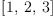(1)
Type: List(PositiveInteger?)
fricas
first(l)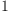(2)
Type: PositiveInteger?
fricas
l2 := rest(l)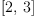(3)
Type: List(PositiveInteger?)
fricas
first(l2)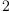(4)
Type: PositiveInteger?
fricas
l3 := rest(l2)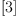(5)
Type: List(PositiveInteger?)
fricas
empty?(l3)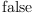(6)
Type: Boolean
fricas
empty?(rest(l3))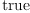(7)
Type: Boolean

One can use elt to access value in arbitrary node effectively using list as an array. But usually this is inefficient, because such access to N-th node has to traverse all preceding nodes, giving O(N) operation. Note: some languages do not have true lists, they use arrays but call them lists. Array access is constant cost operation, use them if you need quick access to an arbitrary elements and do not need flexibility given by lists.

One of most common operations of lists is creating list from sequence of values. One can create list with given value and given tail by using cons. Several applications of cons can create any (proper) list. For example:

fricas
l := empty()$List(Integer)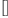(8) Type: List(Integer) fricas l := cons(3, l)(9) Type: List(Integer) fricas l := cons(2, l)(10) Type: List(Integer) fricas l := cons(1, l)(11) Type: List(Integer) Of course, in sequential code square brace notation '[1, 2, 3]?' is much more convenient. But cons can be used in loops: fricas l := empty()$List(Integer)(12)
Type: List(Integer)
fricas
for i in 1..10 repeat l := cons(i, l)
Type: Void
fricas
l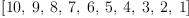(13)
Type: List(Integer)

Note that in both examples elements appear in opposite order to applying 'cons': the last application of cons creates first node of new list. We could correct this problem by using concat, but that is potentially inefficient since concat has to copy the whole list leading to quadratic algorithm (concat! avoids copy but still using it is quadratic due to need to traverse preceding nodes). Instead, the standard idiom is to reverse the list after construction. In normal case after construction the freshly build list in reverse order is no longer needed, so we can use reverse! which reuses nodes to make reversal faster:

fricas
l := reverse!(l)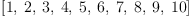(14)
Type: List(Integer)

Note: after calling reverse! old value stored in l is no longer useful, we have to use value returned by reverse!.

We frequently need to create new list by applying the same transformation to all elements of list. FriCAS has special notation in this case:

fricas
l2 := [i^2 for i in l]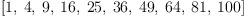(15)
Type: List(Integer)

Note: instead of i^2 we can use more complicated expression and we can replace for part by other FriCAS loop controls. For more details see FriCAS book, chapter 5.4 "Loops" and 5.5 "Creating lists and streams with iterators""). Here we just note that this lead to very efficient code (slightly more efficient that construction in reverse order followed by reversal).

Parts of list can be shared:

fricas
l1 := [1, 2, 3](16)
Type: List(PositiveInteger?)
fricas
l2 := cons(10, l1)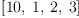(17)
Type: List(PositiveInteger?)
fricas
l3 := cons(20, l1)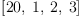(18)
Type: List(PositiveInteger?)

l2 has on "own" node and other nodes are shared with l1 and l3. Similarly l3 has one "own" node and the other are shared. One can see that nodes are shared by modifying them:

fricas
setfirst!(l1, 15)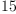(19)
Type: PositiveInteger?
fricas
l1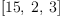(20)
Type: List(PositiveInteger?)
fricas
l2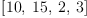(21)
Type: List(PositiveInteger?)
fricas
l3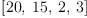(22)
Type: List(PositiveInteger?)

Above we used modification on to show sharing. However in general modifying shared structures may give tricky results. So normal practice is to avoid modifying shared lists. In other words we first build list which may use modification (notably reversing list in place), then we use it without further changes.

When Spad functions modify lists there is fundamental limitation: at low level lists are represented by pointers and called function has copy of a pointer to the list. So function can modify list elements, but can not change pointer in the caller. In other words, function can shorten a list, but can not change nonempty list to empty list:

fricas
fun1(l) ==
empty?(l) => l
l := []
l
Type: Void
fricas
l := [1, 2, 3](23)
Type: List(PositiveInteger?)
fricas
fun1(l)
fricas
Compiling function fun1 with type List(PositiveInteger) -> List(
PositiveInteger)(24)
Type: List(PositiveInteger?)
fricas
l(25)
Type: List(PositiveInteger?)

To avoid this limitation usual convention is that caller should use return value of a function:

fricas
l := reverse!(l)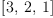(26)
Type: List(PositiveInteger?)
fricas
l(27)
Type: List(PositiveInteger?)
fricas
l := fun1(l)(28)
Type: List(PositiveInteger?)
fricas
l(29)
Type: List(PositiveInteger?)

Note that after modification value of original list may be useless, while return value is OK. For example

fricas
bb := [3, 4, 1, 2]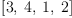(30)
Type: List(PositiveInteger?)
fricas
bs := sort! bb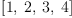(31)
Type: List(PositiveInteger?)
fricas
bb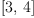(32)
Type: List(PositiveInteger?)
fricas
bs(33)
Type: List(PositiveInteger?)

sort! rearranges connections between list nodes, so orignal list looks too short. But return value is correct. So remember to use return value!

If one really wants to change argument there is a trick of prepending dummy element:

fricas
fun2(l) ==
t := l
t := []
setrest!(l, t)
Type: Void
fricas
l := [1, 2, 3](34)
Type: List(PositiveInteger?)
fricas
dl := cons(0, l)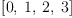(35)
Type: List(Integer)
fricas
fun2(dl)
fricas
Compiling function fun2 with type List(Integer) -> List(Integer)(36)
Type: List(Integer)
fricas
rest(dl)(37)
Type: List(Integer)

Note: we need variable t and extra assignment to t to work around weakness of interpreter. We should be able to use '[]' directly as the second argument to setrest!.

 Subject:   Be Bold !! ( 14 subscribers )